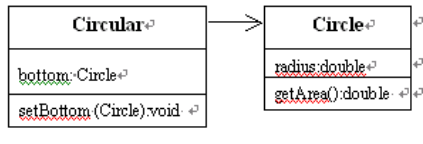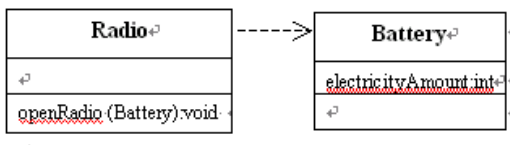# 变量声明

``````class 类名{
//类体内容：
//变量声明:
成员变量=实例变量=实例=实例成员
static 成员变量=类变量=静态变量

//方法的定义:
实例方法=[修饰符] 类型 方法名称1(参数|也是局部变量){
构造函数=方法名称1()
局部变量
}
类方法=static 实例方法=static 类型 方法名称2(参数|也是局部变量){
构造函数=方法名称2()
局部变量
}
}
``````

## 成员变量与局部变量

成员变量 局部变量

static修饰时 类变量、静态变量

1. 参数–整个方法有效
2.方法中定义–在方法中且方法中声明位置之后有效
3.局部变量声明在复合语句中–在该复合语句内且在声明位置之后有效

### 有效性–局部优先

``````class Tom {
int x = 10,y;
void f() {
int x = 5;
y = x+x;
//y得到的值是10，不是20。
//如果方法f 中没有“int x=5;”，y的值将是20
}
}
//------------------------------
class Tom {
int x = 10,y;
void f() {
int x = 5;
y = x+this.x;    //y得到的值是15
}}    //this当前所在区域

``````
``````public class test {
int a = 10, b = 0;              //成员变量，在整个类中有效
public static void main(String[] args) {
//m = 9;                    //非法,因为m声明位置在后面,声明位置之后才有效
int m = 10, sum = 0;        //局部变量，在函数中且在声明位置之后有效
if (m > 9) {
//    z = 2 * m + z;    //非法,因为z声明位置在后面,声明位置之后才有效
int z = 10;             //z仅仅在该复合语句后面有效
z = 2 * m + z;          //合法，局部变量，在复合语句中且在声明位置之后有效
}
for (int i = 0; i < m; i++) {
sum = sum + i;          //合法，i仅仅在该循环语句中有效
}
m = sum;                    //合法，因为m和sum有效
//   z = i+sum;             //非法，因为i和z已无效
}
}
``````

### 默认值

``````//例如:下列InitError类无法通过编译。

class InitError {
int x = 10,y;      //y的默认值是0
void f() {
int m;         //m没有默认值，但编译无错误
x = y+m;    //无法通过编译，因为在使用m之前未指定m的值
}
}
``````

``````public class DefaultValue {
// 实例成员变量
private boolean bool;
private byte b;
private short s;
private char c;
private int i;
private long l;
private float f;
private double d;
private String str;
private String[] strArray;
// 静态成员变量
private static boolean boolStatic;
private static byte bStatic;
private static short sStatic;
private static char cStatic;
private static int iStatic;
private static long lStatic;
private static float fStatic;
private static double dStatic;
private static String strStatic;
private static String[] strArrayStatic;

public void printInstanceField() {
System.out.println("实例成员变量默认值：");
System.out.println("boolean:" + bool);
System.out.println("byte:" + b);
System.out.println("short:" + s);
System.out.println("char:" + c);
System.out.println("int:" + i);
System.out.println("long:" + l);
System.out.println("float:" + f);
System.out.println("double:" + d);
System.out.println("String:" + str);
System.out.println("String[]:" + strArray);
}

public static void printStaticField() {
System.out.println("静态成员变量默认值：");
System.out.println("boolean:" + boolStatic);
System.out.println("byte:" + bStatic);
System.out.println("short:" + sStatic);
System.out.println("char:" + cStatic);
System.out.println("int:" + iStatic);
System.out.println("long:" + lStatic);
System.out.println("float:" + fStatic);
System.out.println("double:" + dStatic);
System.out.println("String:" + strStatic);
System.out.println("String[]:" + strArrayStatic);
}

public static void main(String[] args) {
DefaultValue dv = new DefaultValue();
dv.printInstanceField();
dv.printStaticField();
}
}
/*

boolean:false
byte:0
short:0
char:
int:0
long:0
float:0.0
double:0.0
String:null
String[]:null

boolean:false
byte:0
short:0
char:
int:0
long:0
float:0.0
double:0.0
String:null
String[]:null
*/

``````
``````public class CharDefaultValue {
static char c;

public static void main(String[] args) {
System.out.println((int) c);
System.out.println(c == '\u0000');
}
}
/*
0
true
*/
``````

`````` public static void main(String[] args) {
int[] value = new int;
System.out.println(value);
}
//0
``````

## 对象的组合与复用

（1）通过组合对象来复用方法也称“黑盒”复用，因为当前对象只能委托所包含的对象调用其方法，这样一来，当前对象对所包含的对象的方法的细节（算法的细节）是一无所知的。

（2）当前对象随时可以更换所包含的对象，即对象与所包含的对象属于弱耦合关系。

``````class b{
getFunb{

}
}
//-------------------
class A{
B b;        //B是类，b是b声明的对象，类A把对象b作为自己的成员变量
setFuna(B temp){
b = temp;
}
getFuna(){
return getFunb()*2; //getFuna()函数调用getFunb函数
}
}
//--------------------
A a;            //类A声明了对象a,此时a的只是一个引用，其他的什么也没有
//--------------------
a = new A();    //声明的对象a分配变量,此时a成员变量已经有了默认值，a的b是引用型变量，默认值是null
//--------------------
B b1 = new B(); //B声明了一个对象b1，并为其分配变量，此时b1是一个引用，并且指向一个空间
/*（对象的本质就是变量，类数据类型和int一样，对象引用型变量，和int test 的test一样，只不过是个引用型变量，对比为数组，int test1[],test1和上面的b1一样，指向一个空间，自身是个引用变量，类似指针）*/
//--------------------
a.setFuna(b1);		//a调用a的函数，将b1的引用传给a的b，此时b1和a的b拥有相同引用，指向相同的空间，且a的b可以委托调用B的方法
//--------------------
B b1 = new B(); 	//此时对A的a没有任何影响，只是b1重新指向别的空间了
//-------------------
a.getFuna();		//a调用a的getFuna()函数，其中getFuna()函数调用getFunb函数

``````

### 关联关系和依赖关系

A中的成员变量是用B类声明的对象，那么A和B的关系是关联关系，称A类的对象关联于（或组合了）B类的对象